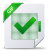# LUA Images Arithmetic Operations

## Cours LUA Images Arithmetic Operations, tutoriel & guide de travaux pratiques en pdf.

Image library methods

number image.getfiletype(filename)
Orders FreeImage to analyze the bitmap signature. The function then re-turns one of the predefined FREE IMAGE FORMAT constants or a bitmap identification number registered by a plugin. Because not all formats can be identified by their header, filename extension are used to determinate the file format.
number image.getfiletypebyext(filename)
Return the predefined FREE IMAGE FORMAT constants looking only the filename extension.
Image image.load(filename, FormatID, flag = 0)
This function decodes a bitmap, allocates memory for it and then returns it as a Image (Lua UserData/Object). A parameter defines the type of bitmap to be loaded. For example, when FIF BMP is passed, a BMP file is loaded into memory (an overview of possible FREE IMAGE FORMAT constants is available in Constants section). Optional Flag (default is 0) can be used to change some load specific behaviour.
Try to load image and use getfiletype to determinate the image type.
Image image.new(width, height, bpp)
Create new image width specified width,height and bpp. Only bpp 8,24,32 are currently supported. Return a LUA userdata Image Object.

### Images Arithmetic Operations

Sum (clamping) two images
Image image.sub(a,b)
Subtract (clamping) two images
Image image.lightest(a,b)
Return lightest pixels of two images
Image image.darkest(a,b)
Return darkest pixels of two images
Image image.avg(a,b)
Return mix of the images
Image image.blend(a,b,perc)
Mix image of the images using perc factor (0.0 .. 1.0)

#### Image Methods

Image:save(filename, FormatID, flag = 0)
Save image onto filename with FormatID file format (see Costants). Flag (0 used as default) can used to specify image quality.
Image:save(filename)
Save image onto filename using format returned by getfiletypebyext
number Image:width()
number Image:height()
Returns the width,height of the bitmap in pixel units.
number Image:bpp()
Returns the size of one pixel in the bitmap in bits. For example when each pixel takes 32-bits of space in the bitmap, this function returns 32. Possible bit depths are 1, 4, 8, 16, 24, 32 for standard bitmaps and 16-, 32-, 48-, 64-, 96- and 128-bit for non standard bitmaps.
Image Image:rescale(width, height, FilterMethod)
Rescale object to width and height, using FilterMethod (see Costants).
Image Image:convert(bpp)
Change bpp of object to 8,24,32.
Image image:clone()
Makes an exact reproduction of an existing bitmap, including metadata and attached profile if any.
Image Image:crop(x0,y0,x1,y1)
Image Image:paste(x0,y0)
Image Image:rotateclassic(angle)
Image Image:blur(var)
Apply a gaussian blur on the image
Image:flip()
Flip vertically the image
Image:mirror()
Flip horizzontally the image
Image:invert()
Invert colors in image
Image:binarize(th)
Binarize image (0 under the threshold, 255 over the threshold)
Image:quantize(levels)
Quantize the channel to n levels
Image:brightness(factor)
Multiply any pixel value by f actor.
Image:wnoise(factor)
Change image gamma correction
Image:merge(b,m)
Mix current image with image b using mask image m

Drawing API

Image:color(grey)
Image:color(grey,alpha)
Image:color(r,g,b)
Image:color(r,g,b,alpha)
Set the current drawing color
Image:box(x0,y0,x1,y1)
Draw a filled box using drawing color
Image:clear()
Reset entire image using drawing color
Image:rectangle(x0,y0,x1,y1)
Draw a rectangle (frame)
Image:line(x0,y0,x1,y1)
Draw a line
Image:circle(x,y,r,filled)
Draw a circle
Image:ellipse(x0,y0,x1,y1,filled)
Draw an ellipse
Image:pixel(x,y)
Draw a point using current color.
Image:pixel(x,y, grey)
Image:pixel(x,y, grey,alpha)
Image:pixel(x,y, r,g,b)
Image:pixel(x,y, r,g,b,alpha)
Draw a point using specified color.
(r,g,b,a) = Image:value(x,y)
Return the pixel valueTélécharger le cours complet